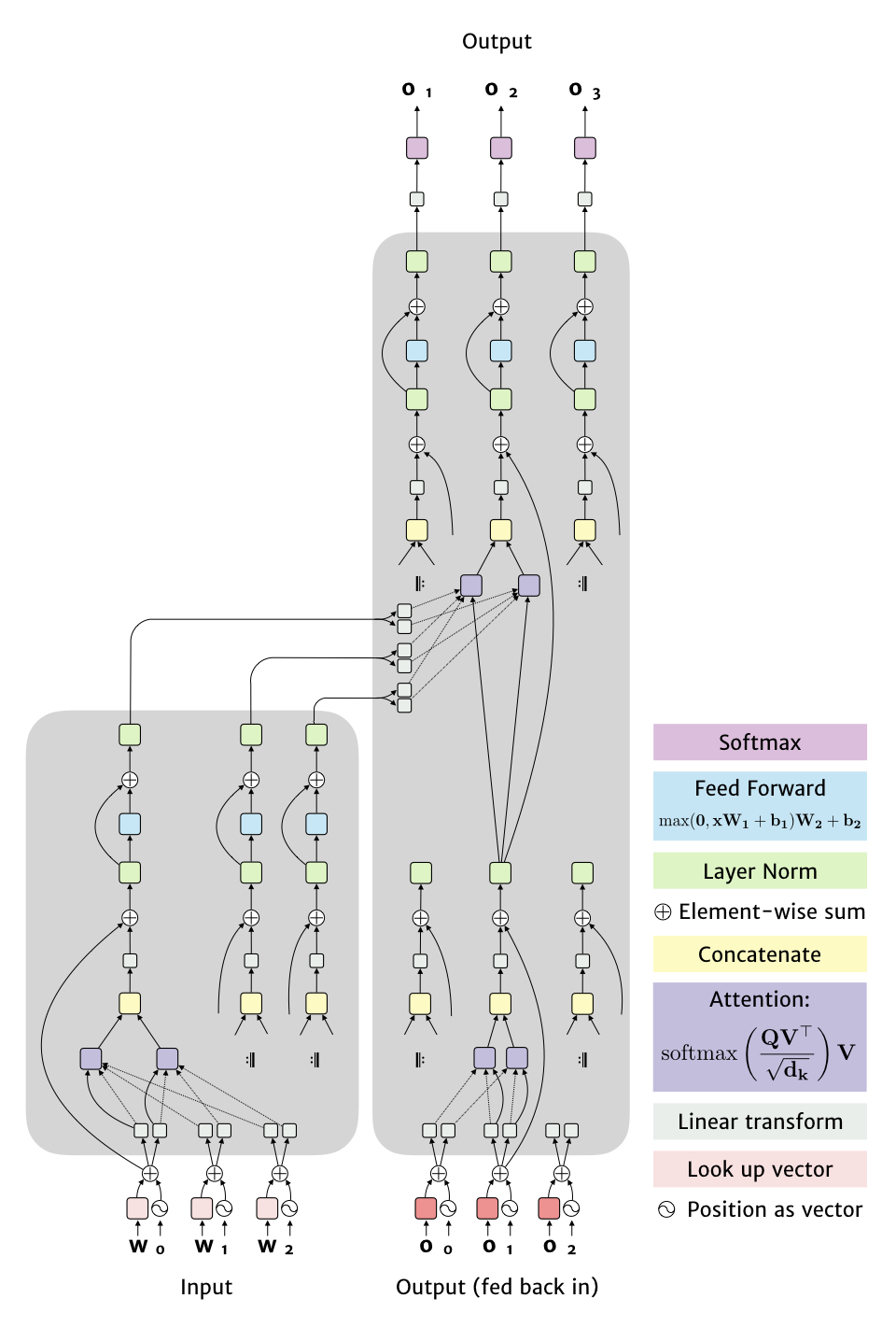# Attention Is All You Need (Vaswani et al., ArXiv 2017)

To get context-dependence without recurrence we can use a network that applies attention multiple times over both input and output (as it is generated).

Recurrent neural networks like LSTMs and GRUs have limited scope for parallelisation because each step depends on the one before it. This architecture also means that many steps of computation separate two words that are far apart, making it difficult to capture long-distance relations. A range of approaches have been used to try to address these issues, such as convolutional structures and other forms of recurrence (e.g. QRNNs). The idea in this work is to use attention, applied multiple times, to get a network that is fast while still capturing positional information.

To explain the structure I put together the figure below, which captures the network structure with a few simplifications:There are a few ideas being brought together here:

• Positional encoding, which is a vector of the same length as the word representation, but that depends only on the position in the input. Here they use $f(pos, dim) = sin(pos / 10000^{2 dim / d_w})$ for even dimensions and the cosine equivalent for odd dimensions (where $d_w$ is the number of dimensions.
• Multi-head attention, where rather than running attention once on the full vector, multiple linear transforms are applied to get smaller vectors.
• Scaled dot product attention, the equation is shown in the figure, the key new idea is to rescale by the square root of the dimensionality so that larger vectors don’t produce excessively sharp distributions after the softmax is applied. The more general form of this described in the paper has keys ($K$), queries ($Q$) and values ($V$), but the network uses the same vector for the key and value. I show the query with a solid line and the values/keys with dotted lines. The matrix $V$ is formed by using the $v$ vectors as rows, while $Q$ is formed by duplicating $q$ in every row. Note, on the left hand side attention is over all input words, while on the right it is only over the words produced so far (ie. content to the left).
• Layer normalisation, a way to rescale weights to keep vector outputs in a nice range, from Ba, Kiros and Hinton (ArXiv 2016).
• Other details, (1) When the outputs are words, the vectors used to initially represent each input word are also used to represent the outputs and in the final linear transformation (though with some rescaling). (2) They use a formula I haven’t seen before to adjust the learning rate during training, (3) dropout in several places and label smoothing are used for regularization.

Simplifications in the figure:

• For multi-head attention I only show two transforms, while in practise they used 8.
• The shaded regions are duplicated 6 times to create a stack, with the output of one region acting as the input to the next copy of it. The links from left to right are always from the top of the input stack.
• The musical repeat signs indicate that the structure is essentially the same. On the output side this isn’t quite true since the attention boxes only take inputs to their left (since output to the right doesn’t exist when they are being calculated).

In terms of experiments, it works at least as well if not better than prior approaches, and is a lot faster for machine translation (no speed numbers are given for parsing). There is also some nice analysis of what it ends out using the attention mechanism to focus on for each word. It seems like it can provide a way to effectively disambiguate the sense of a word based on its context.

## Citation

ArXiv Paper

Google also has some blog posts up about the paper and about the library they released.

@article{arxiv:1706.03762,
author    = {Vaswani, Ashish and Shazeer, Noam and Parmar, Niki and Uszkoreit, Jakob and Jones, Llion and Gomez, Aidan N. and Kaiser, Lukasz and Polosukhin, Illia},
title     = {Attention Is All You Need},
title: = {Attention Is All You Need},
journal   = {ArXiv},
year      = {2017},
url       = {http://arxiv.org/abs/1706.03762},
}